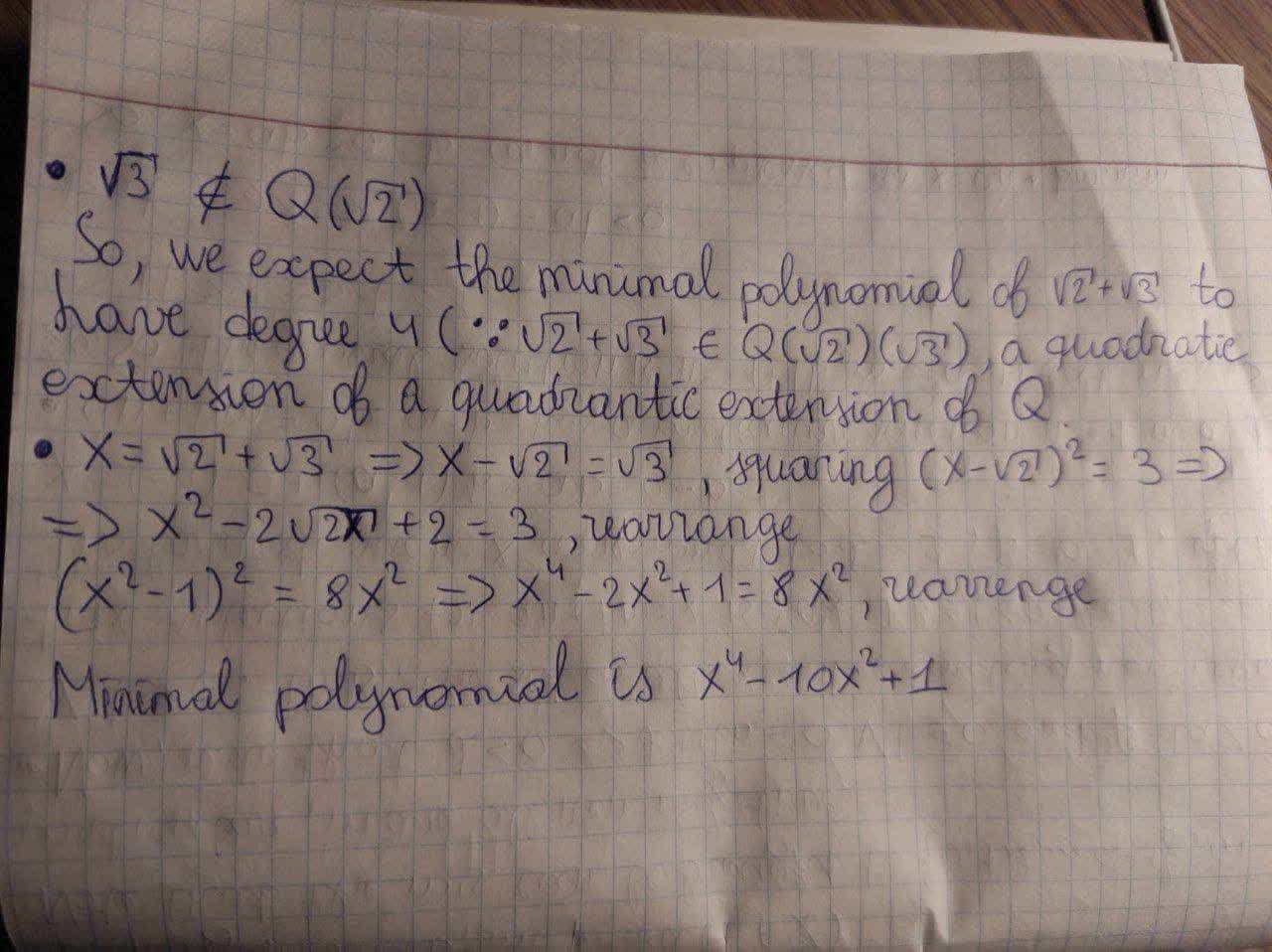# Find th eminimal polynomial of sqrt2+sqrt3 over QQrocedwrp 2020-11-10 Answered
Find th eminimal polynomial of $\sqrt{2}+\sqrt{3}$ over $\mathbb{Q}$
You can still ask an expert for help

• Questions are typically answered in as fast as 30 minutes

Solve your problem for the price of one coffee

• Math expert for every subject
• Pay only if we can solve itJayden-James Duffy
To find the smallest degree polynomial f(x) with coefficients in Q, such that $f\left(\sqrt{2}+\sqrt{3}\right)=0.$
The impo
rtant point is that the coefficients should all be in Q. For example, $x-\left(\sqrt{2}+\sqrt{3}\right)$ is not allowed, as its coefficients are not rational numbers.
Now, $\sqrt{3}\notin \mathbb{Q}\sqrt{2}.$
So, we expect the minimal polynomial of $\sqrt{2}+\sqrt{3}$ to have degree $4\left({,}^{\prime }\sqrt{2}+\sqrt{3}\in \mathbb{Q}\left(\sqrt{2}\right)\cdot \left(\sqrt{3}\right)$, a quadrantic extension of a quadrantic extension of $\mathbb{Q}$.
We proceed to find this minimal polynomial by repeated squaring (to clear radicals and obtain rational coefficients)
$x=\sqrt{2}+\sqrt{3}⇒x-\sqrt{2}=\sqrt{3},$ squaring
${\left(x-\sqrt{2}\right)}^{2}=3⇒{x}^{2}-2\sqrt{2}x+2=3$, rearrange
${x}^{2}-1=2\sqrt{2}x$, square again
${\left({x}^{2}-1\right)}^{2}=8{x}^{2}⇒{x}^{4}-2{x}^{2}+1=8{x}^{2}$, rearrange
Minimal polynomial is ${x}^{4}-10{x}^{2}+1$